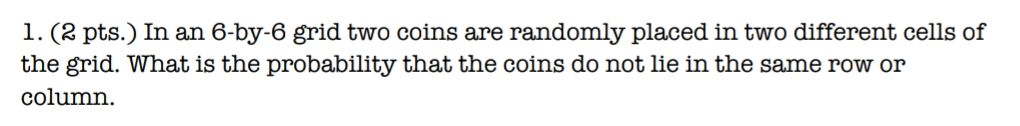### ¿Todavía tienes preguntas de matemáticas?

Pregunte a nuestros tutores expertos
Algebra
Pregunta1. (2 pts.) In an 6-by-6 grid two coins are randomly placed in two different cells of the grid. What is the probability that the coins do not lie in the same row or column.

$$P= \frac{36\times 25}{36\times 35} = \frac{5}{7}$$